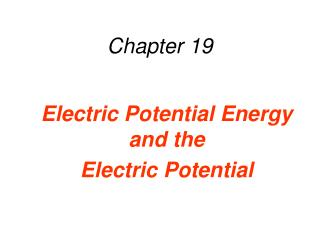DownloadDownload PresentationChapter 19

# Chapter 19

Télécharger la présentation## Chapter 19

- - - - - - - - - - - - - - - - - - - - - - - - - - - E N D - - - - - - - - - - - - - - - - - - - - - - - - - - -
##### Presentation Transcript

1. Chapter 19 Electric Potential Energy and the Electric Potential

2. Calculate the change of PEg for: A 10.0 kg object falling 10.0 m A 5.0 kg object falling 10.0 m Calculate the change of PEg per mass for each object. Make a conclusion: what does this ratio depend on? What does it characterize? Which force is doing work on the ball? Is the work positive or negative? Does it lead to increase or decrease of potential energy?

3. Analogy Which force is doing work? Which energy is changing? Is the energy increasing or decreasing?

4. Analogy Gravitational field’s strength Electric field’s strength What characterizes the object What characterizes the object What characterizes the motion What characterizes the motion

5. Analogy Formula for Formula for

6. White Board Practice Draw a uniform electric field. E = ___________. Make up two positive charges and place them in the field. Let them travel __________ cm. Calculate the change in EPE for each charge. Calculate the ratio

7. DEFINITION OF ELECTRIC POTENTIAL DIFFERENCE Potential difference – ratio of the change of EPE per charge DEFINITION OF ELECTRIC POTENTIAL Potential difference – ratio of EPE per charge SI Unit of Electric Potential: joule/coulomb = volt (V)

8. BACK TO THE BOARD Return the two charges to their original position in the field. Mark that point with a V1 to indicate potential at that point Mark a place 10.0 cm down the direction of the field. Mark it with V2 For each charge, calculate Change of EPE Potential difference between V1 and V2 Compare the magnitudes of V1 and V2 (which one of them is greater) What is the natural direction of a positive charge moving in an electric field: - in the direction of the field / - opposite the direction of the field - towards a lower potential / towards a higher potential

9. White Board Practice In the center of the field, place a positive and a negative charge (make up their magnitudes). Show the direction of the electric force on each charge. Allow the force move the charge 5.0 cm. Calculate the change of EPE for each charge. Calculate potential difference that each charge naturally went through.

10. Conclusion: EPE characterizes _________________________ (charge or field) Potential characterizes ______________________ (charge or field) ______________________ force works on charges as they move in EF As charges move in an electric field naturally, their EPE _______________ If EPE increases, __________________ force has worked on the charge Positive charges naturally move towards ___________________ potential Negative charges move towards _____________________ potential.

11. Review Charges have _________________________ associated with their position in an ____________________________ Charges that are moving naturally in an ____________________, lose / gain _______________________________ _____________________ characterizes the charge Ratio of __________________ per charge is called _____________________ Ratio of ___________________ per charge is called __________________________________________________ Positive charge naturally moves through a _____________________________ Negative charge naturally moves through a ____________________________

12. The Accelerations of Positive and Negative Charges A positive test charge is released from A and accelerates towards B. Upon reaching B, the test charge continues to accelerate toward C. Assuming that only motion along the line is possible, what will a negative test charge do when released from rest at B?

13. Checkyourunderstanding + + A +5.0 nC charge moves 5.0 cm at a 60-degree angle to the direction of the field. Calculate the change in its potential energy.

14. Practice problems How far should a proton move in a UEF, E = 3000 V/m to move through a potential difference = 60 V? How far should an electron move in the same EF to move through 60V of potential difference? Which particle will lose EPE?

15. Practice Problems A 15.0 g particle carrying 2.0x109 extra electrons is released in a 5000 V/m UEF directed to the right Which way will the particle move? How far will it have moved when it’s EPE drops by 160 J? What potential difference will it have moved through? (find it using 2 methods) What will be the speed of the particle at that moment? (Hint: conservation of mechanical energy)

16. Practice problems A negative particle with a charge of - 5.0 nC and a mass of 5.0 g is released in a UEF directed to the right. Determine the strength of the electric field if the horizontal speed of the particle is 15 m/s after travelling 25 cm.

17. The Conservation of Energy A particle has a mass of 1.8x10-5kg and a charge of +3.0x10-5C. It is released from point A and accelerates horizontally until it reaches point B. The only force acting on the particle is the electric force, and the electric potential at A is 25V greater than at C. (a) What is the speed of the particle at point B? (b) If the same particle had a negative charge and were released from point B, what would be its speed at A?

18. Unit Play